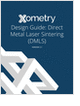Formula HomeFinanceFinancial TermsMoney FactorBackgroundCalculatorsLoan (typical)Loan (advanced)Canadian MortgageLease (typical)Lease (advanced)APR (typical)APR (advanced)ResourcesBibliography
Metal 3D Printing Design Guide

Direct Metal Laser Sintering (DMLS) 3D printing for parts with reduced cost and little waste.

Mechanical Engineers Outlook

Guide for those interested in becoming a mechanical engineer. Includes qualifications, pay, and job duties.

3D Scanners

A white paper to assist in the evaluation of 3D scanning hardware solutions.

STEM Career Outlook

Wages, employment opportunities, and growth projections for STEM jobs.

more free publicationsMoney Factor DefinitionRecall that the monthly payment for a lease is given by,where C is the loan amount, F is the residual value, r is the monthly interest rate, and N is the term of the lease. The interest part of the monthly payment of the ith month can be written as,For typical auto lease terms (interest rate < 15% and term < 60 months), the plot of interest payment is very smooth and approaches a straight line1:The total interest payment in N months is represented by the shaded area which can be approximated by the area of the trapezoid defined by the dotted line,Note that the Total Interest calculated this way is slightly lower since the trapezoid is always smaller than the shaded area. The monthly payment of a lease can therefore be estimated by adding the average principal payment and the average interest payment,where Money Factor = r/2. Alternatively, if the annual interest rate is R%, then the Money Factor is equal to R/2400.Important: When a car dealer quotes you a Money Factor, you can always multiply that by 2400 to get a very good feeling about the actual interest rate %. Also, the monthly payment calculated by the Money Factor is always slightly lower than that calculated by the 'real' formula. For example, if a dealer tells you his lease Money Factor is .0025, then you simply multiply that by 2400 to get 6. Now you know that the actual interest rate behind this Money Factor is slightly below 6%. Sometimes a dealer will give you a Money Factor like 3.1 so it sounds like a low interest rate. In this case you just multiply that number by 2.4 and immediately know that the interest rate is about 7.44%.1. The straight line approximation relies on a small Nr. See the mathematical proof.
GlossaryMetal 3D Printing Design Guide

Direct Metal Laser Sintering (DMLS) 3D printing for parts with reduced cost and little waste.Mechanical Engineers Outlook

Guide for those interested in becoming a mechanical engineer. Includes qualifications, pay, and job duties.3D Scanners

A white paper to assist in the evaluation of 3D scanning hardware solutions.STEM Career Outlook

Wages, employment opportunities, and growth projections for STEM jobs.